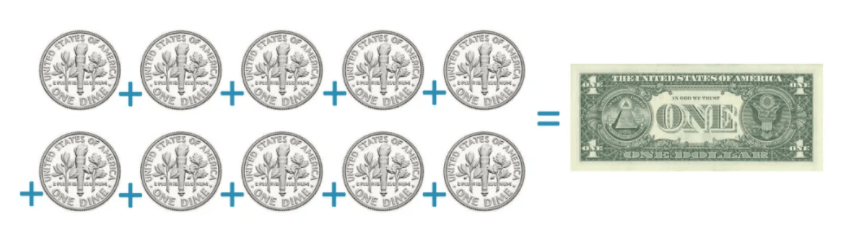# Counting US Coins: Dimes, Nickels, Pennies & Quarters

Counting US Coins: Dimes, Nickels, Pennies & Quarters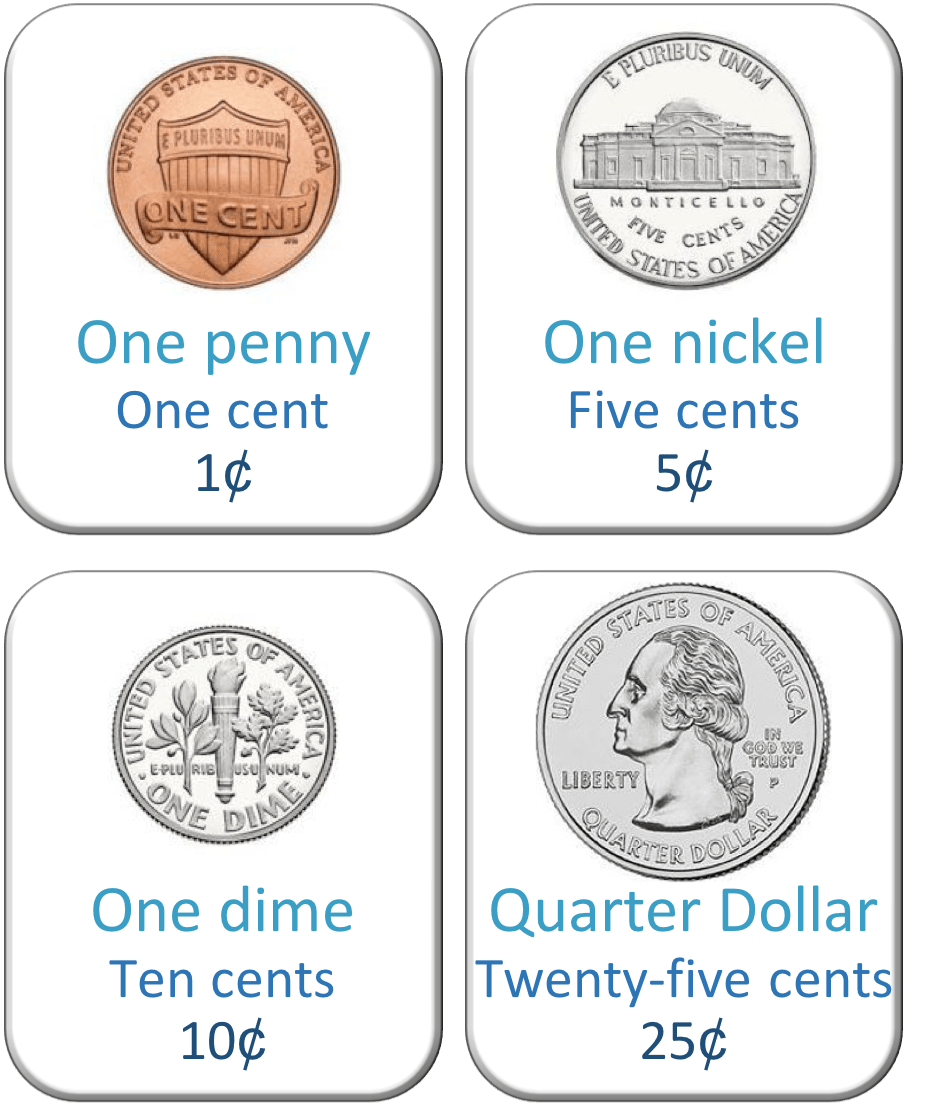• This poster shows the values of the four common coins used in America.
• The value of each coin is based on how many cents it is worth.
• The Penny is worth 1 cent.
• The Nickel is worth 5 cents.
• The Dime is worth 10 cents.
• The Quarter is worth 25 cents.

There are four main American coins: the penny, the nickel, the dime and the quarter.• We have the following American coins: one quarter, one nickel, one dime and three one penny coins.
• One quarter is worth 25 cents.
• One nickel is worth 5 cents.
• We can add these two coins to make a multiple of ten. 25 + 5 = 30 cents.
• One dime is worth 10 cents. We add this coin to our total so far.
• 30 + 10 = 40 cents.
• We have three one penny coins left to add to our total.
• The total of the coins is 40 + 3 = 43 cents.# Values of Pennies, Nickels, Dimes and Quarters

## Value of a Penny Coin

The penny coin is also known as the one-cent coin. The penny coin is worth one cent and it is the American coin of lowest value. One hundred cent coins make a dollar.

Here is a picture of the one-cent penny coin.The cent symbol is ‘¢’ and so we can write one cent as 1¢.

One hundred cents make one dollar.

In dollars, one cent is written $0.01. ## Value of a Nickel Coin The nickel is an American coin that is worth 5 cents. A nickel is worth 5¢ or$0.05.

Here is a picture of a nickel coin.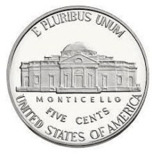The President Thomas Jefferson is the man on the nickel.

One nickel coin is worth the same as five 1 cent coins.A nickel is worth 5¢, which can also be written in dollars as $0.05. Because one hundred cents make a dollar and 20 × 5 = 100, there are 20 nickels in a dollar.## Value of a Dime Coin A dime is worth 10 cents. A dime is worth 10¢, which can be written in dollars as$0.10. Ten dimes are worth one dollar.

Here is a picture of a dime.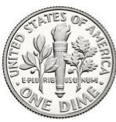The dime coin is worth the same as ten one cent coins.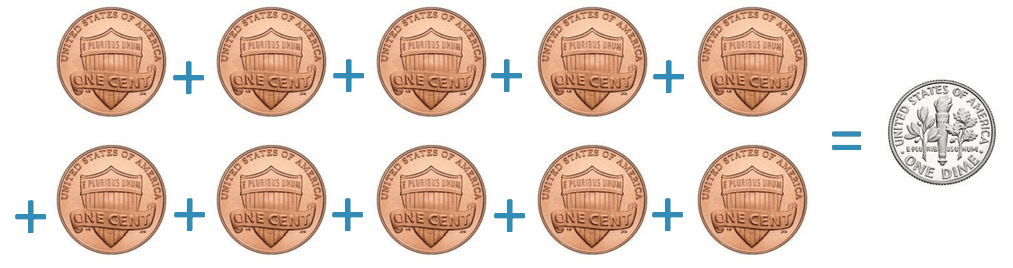A dime is worth 10 cents and a nickel is worth 5 cents. Therefore two nickels are worth the same as one dime.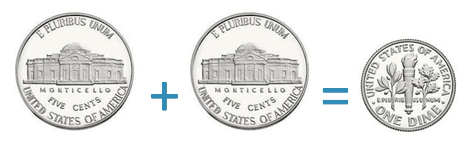Both the nickel and dime coins are silver in colour but the main difference between them is the size. Although the dime is worth more, it is smaller than the nickel coin.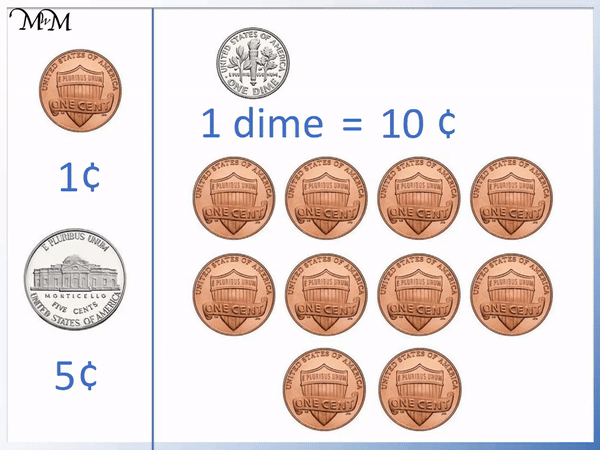## Value of a Quarter Dollar Coin

The quarter is an American coin worth 25 cents. The quarter is worth 25¢, which can be written in dollars as \$0.25. Four quarters are worth one dollar.

Here is a picture of the quarter dollar coin.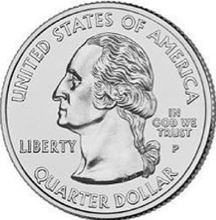A quarter dollar is worth the same as 25 one cent coins.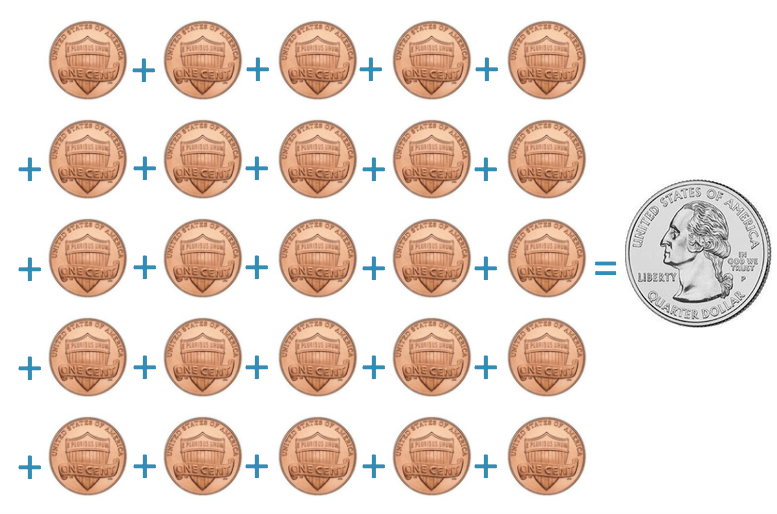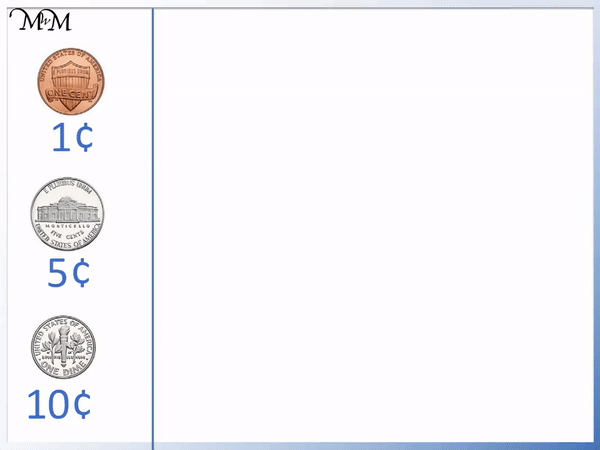A quarter is worth 25¢ and can be made from any combination of coins that add up to make 25 cents.

For example, a quarter dollar can be made from two dimes plus one nickel.

Each dime is worth 10¢ and the nickel is worth 5¢.

10 + 10 + 5 = 25 and so two dimes plus a nickel add up to make 25¢.Starting with 25 one cent coins, we can see that ten one cent coins can be replaced with a dime and five one cent coins can be replaced with a nickel.## Counting American Coins

We can use the values of the individual US coins to help us work out the totals of different combinations of coins.

We just need to remember the following values:

• A one cent coin is worth 1¢
• A nickel is worth 5¢
• A dime is worth 10¢
• A quarter is worth 25¢

In this example we have a quarter, a dime, a nickel and three one cent coins.We have a quarter which is worth 25¢.

We try to add coins to make multiples of 10 if we can.

We can add the nickel to the quarter. 5 + 25 = 30 and so 5¢ + 25¢ = 30¢.

We can easily add the dime to this total by adding 10¢.

30 + 10 = 40 and so 30¢ + 10¢ = 40¢.

Finally, we add the cent coins, which are each worth 1¢.

We have 3 one cent coins, worth 3¢ in total.

40 + 3 = 43 and so we have 43¢ in total.Now try our lesson How to Make a Dollar with Coins where we learn the combinations of different American coins that can make a dollar.